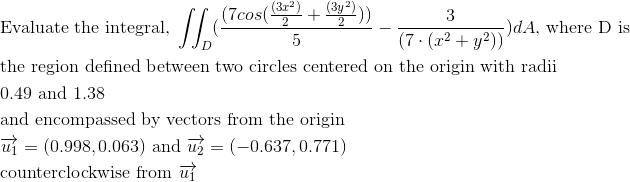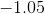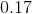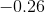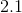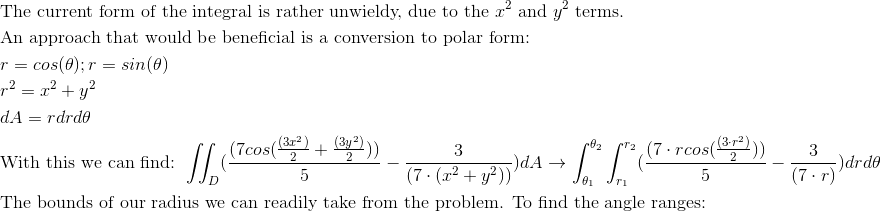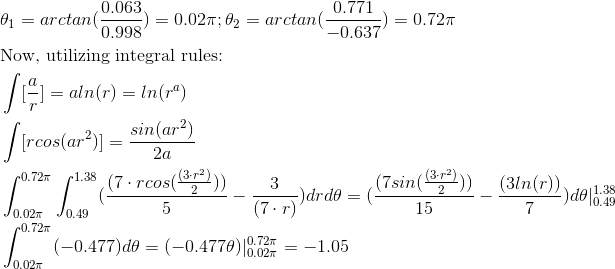Calculus 3 : Double Integrals

Example Questions

Example Question #71 : Double Integration In Polar Coordinates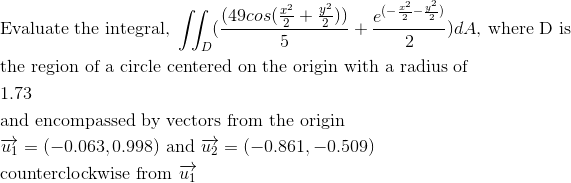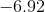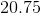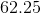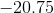Explanation: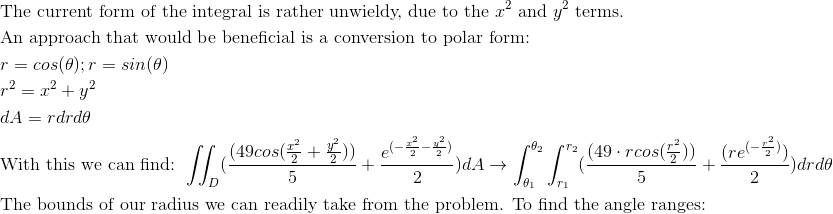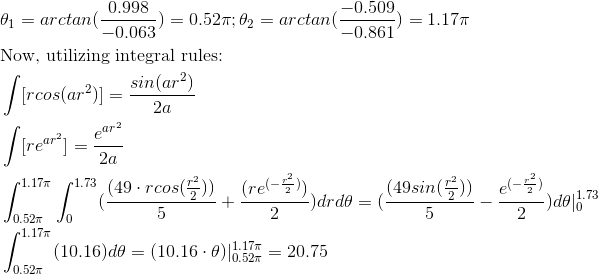Example Question #72 : Double Integration In Polar Coordinates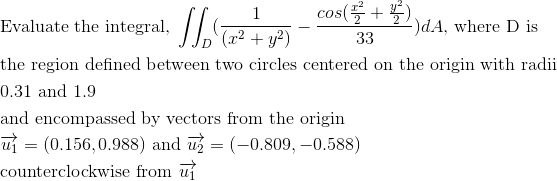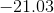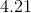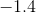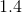Explanation: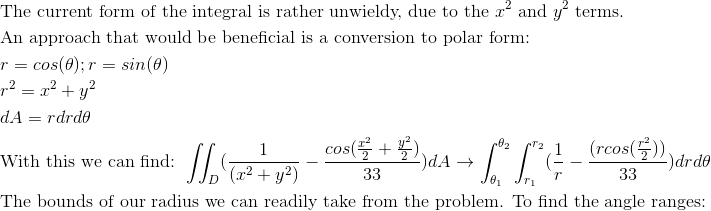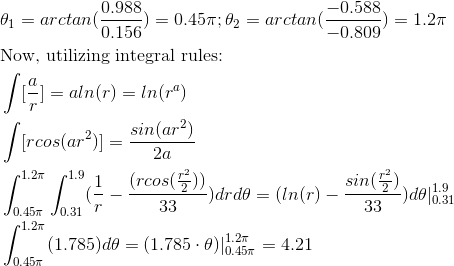Example Question #73 : Double Integration In Polar Coordinates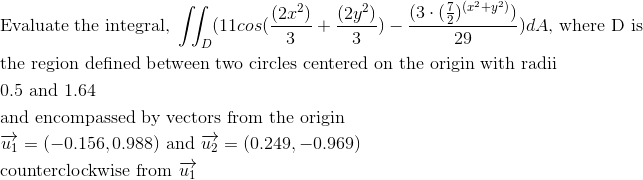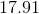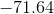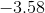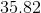Explanation: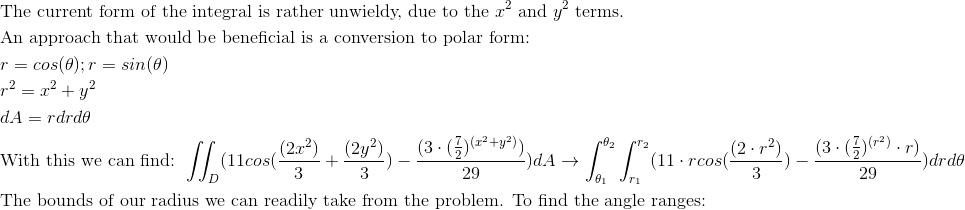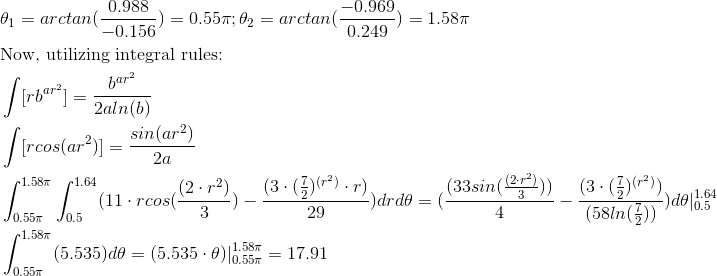Example Question #74 : Double Integration In Polar Coordinates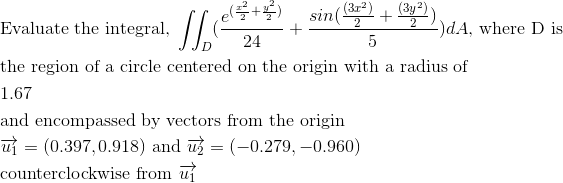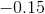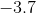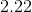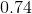Explanation: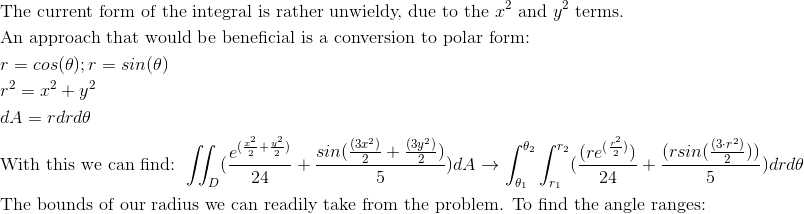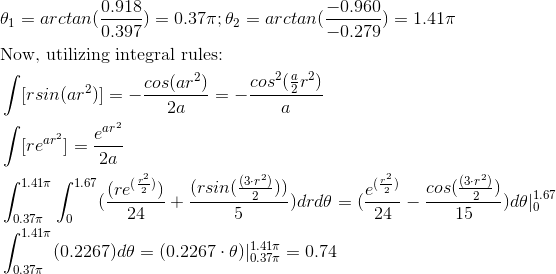Example Question #75 : Double Integration In Polar Coordinates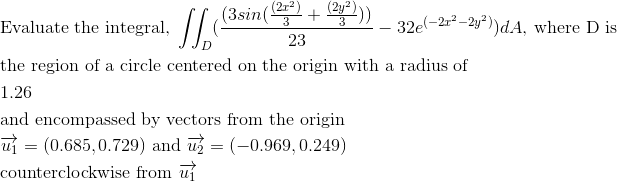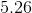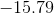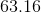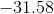Explanation: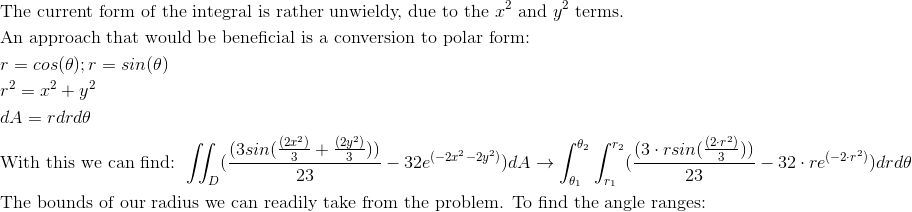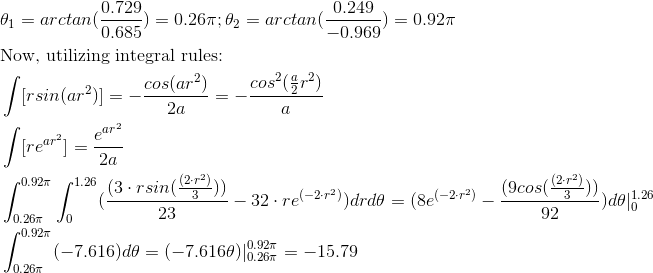Example Question #76 : Double Integration In Polar Coordinates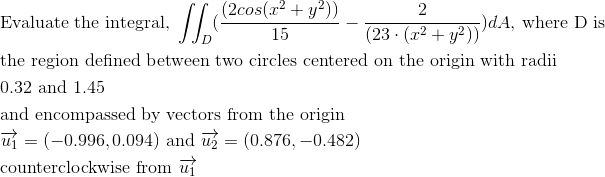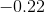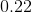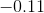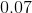Explanation: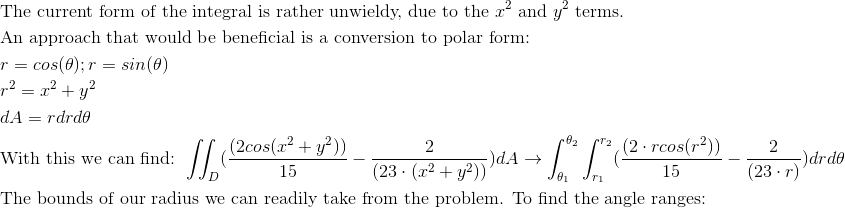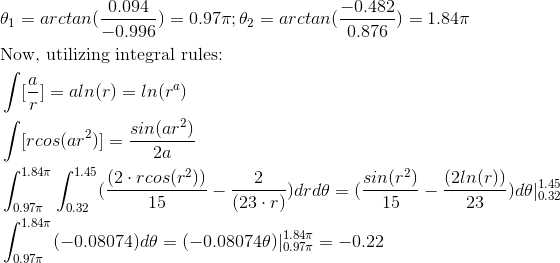Example Question #77 : Double Integration In Polar Coordinates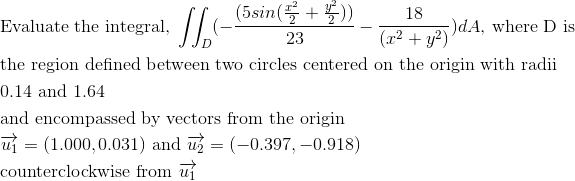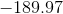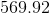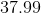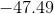Explanation: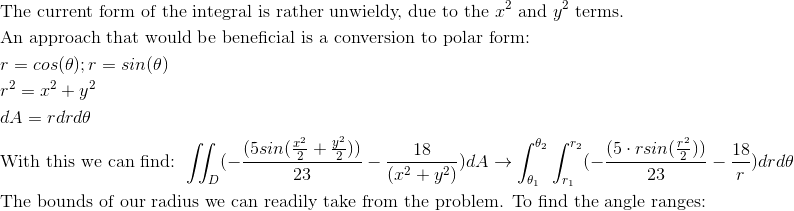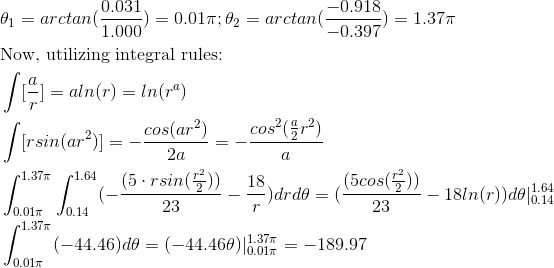Example Question #78 : Double Integration In Polar Coordinates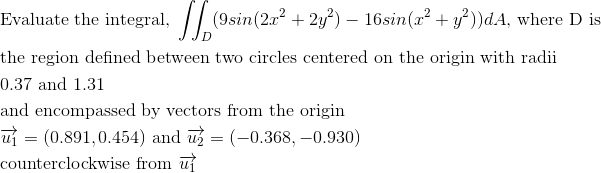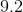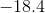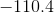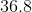Explanation: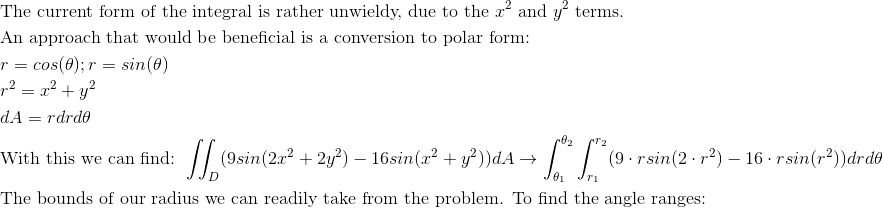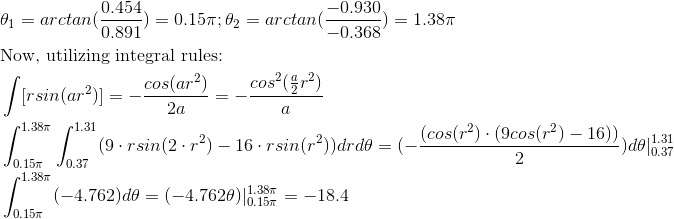Example Question #79 : Double Integration In Polar Coordinates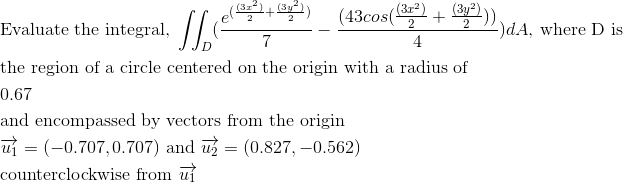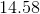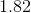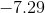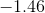Explanation: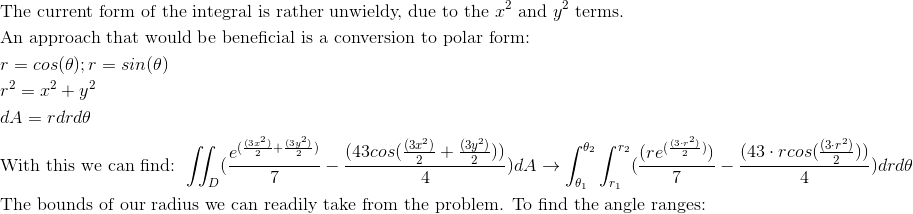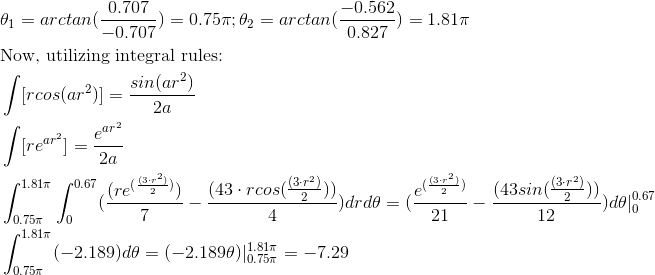Example Question #80 : Double Integration In Polar Coordinates